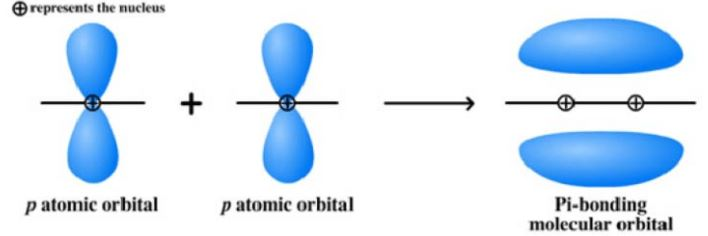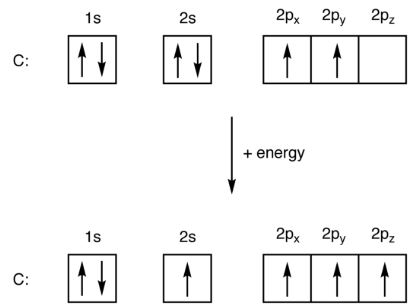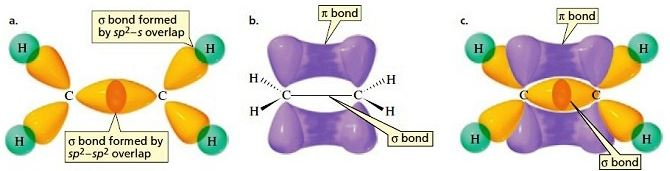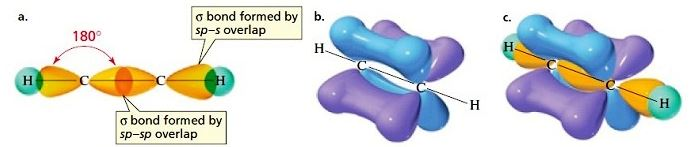Checkout JEE MAINS 2022 Question Paper Analysis : Checkout JEE MAINS 2022 Question Paper Analysis :

# Pi Bonds

## What are Pi Bonds?

Pi bonds are chemical bonds that are covalent in nature and involve the lateral overlapping of two lobes of an atomic orbital with two lobes of another atomic orbital that belongs to a different atom. Pi bonds are often written as ‘𝛑 bonds’, where the Greek letter ‘𝛑’ refers to the similar symmetry of the pi bond and the p orbital.

The two orbitals which are bonded share the same nodal plane at which the electron density is zero. This plane passes through the nuclei of the two bonded atoms and is also the nodal plane for the molecular orbital corresponding to the pi bond.

An illustration depicting the overlapping of two different p orbitals in order to form a 𝛑 bond is given below.Commonly, pi bonding involves p orbitals. However, d orbitals also have the ability to participate in 𝛑 bonding and these types of bonds involving d orbitals can be found in the multiple bonds formed between two metals.

## Examples of Pi Bonding

### Bonding in Ethene (C2H4)

Ethene is often considered the simplest alkene since it contains only 2 carbon atoms (that are doubly bonded to each other) and four hydrogen atoms. The electron configuration of carbon is 1s22s22p2. However, in its excited state, an electron is promoted from the 2s orbital to the 2p orbital.This promotion of a lone pair of 2s electrons occurs when a photon of a specific wavelength transfers energy to the 2s electron, enabling the jump to the 2p orbital. This promotion doesn’t require much energy since the energy gap between the 2s and 2p orbital is very small.

Now, the excited carbon atoms undergo sp2 hybridization to form a sp2 hybridized molecular orbital (which is made up of a single ‘s’ orbital and two ‘p’ orbitals). The sp2 hybridized carbons now form three sigma bonds and one pi bond, as illustrated below.The sp2 hybridized orbital in the carbon atom is made up of a 2s electron, a 2px electron, and a 2py electron. It can form a total of three sigma bonds. The 2pz electrons of the carbon atoms now form a pi bond with each other. Thus, each carbon atom in the ethene molecule participates in three sigma bonds and one pi bond.

### Bonding in Ethyne (C2H2)

Ethene is the simplest alkyne in which each carbon atom is singly bonded to one hydrogen atom and triply bonded to the other carbon atom. These bonds are formed when photons of a specific wavelength transfer energy to the 2s electron, enabling it to jump to the 2pz orbital.

Now, the 2s orbital and one 2p orbital undergo hybridization to form a sp hybridized orbital. This orbital contains the 2s electron and the 2px electron. The 2py and 2pz electrons of the carbon atoms now form pi bonds with each other, as illustrated below.The ‘sp’ hybridized orbital in the carbon atom can form a total of two sigma bonds. One sigma bond is formed with the adjacent carbon atom and the other is formed with the ‘1s’ orbital belonging to the hydrogen atom. Two pi bonds are formed from the lateral overlap of the 2py and 2pz orbitals.

## Strength of Pi Bonds

The pi bonds are almost always weaker than sigma bonds. For example, two times the bond energy of a carbon-carbon single bond (sigma bond) is greater than a carbon-carbon double bond containing one sigma and one pi bond. This observation on the bond strength of 𝛑 bonds suggests that they do not add as much stability as sigma bonds do.

The relative weakness of these bonds, when compared to sigma bonds, can be explained with the help of the quantum mechanical perspective that there is a significantly lower degree of overlapping of p orbitals in pi bonds due to the fact that they are oriented parallel to each other. Sigma bonds, however, have a far greater degree of overlapping and hence tend to be stronger than the corresponding 𝛑 bonds.

## Pi Bonding in Multiple Bonds

The following multiple bonds can be broken down into sigma, pi, and delta bonds:

• Generally, double bonds consist of a single sigma bond and a single pi bond. An example of such a bond can be seen in ethylene (or ethene).
• Typically, triple bonds consist of a single sigma bond and two 𝛑 bonds which are placed in planes that are perpendicular to each other and contain the bond axis.
• Quadruple bonds are very rare bonds which can only be found in the bonds between two transition metal atoms. It can be broken down into one sigma, two pi, and one delta bond.

The combination of pi and sigma bonds in multiple bonds are always stronger than a single sigma bond. The reduction in bond lengths in multiple bonds points towards this statement. This contraction in the bond length can be observed in the length of the carbon-carbon bond in ethane, ethylene, and acetylene, which are equal to 153.51 pm, 133.9 pm, and 120.3 pm respectively.

Therefore, it can be understood that multiple bonds shorten the total bond length and strengthen the overall bond between the two atoms. To learn more about sigma and pi bonds, register with BYJU’S and download the mobile application on your smartphone.

Test your knowledge on pi bonds